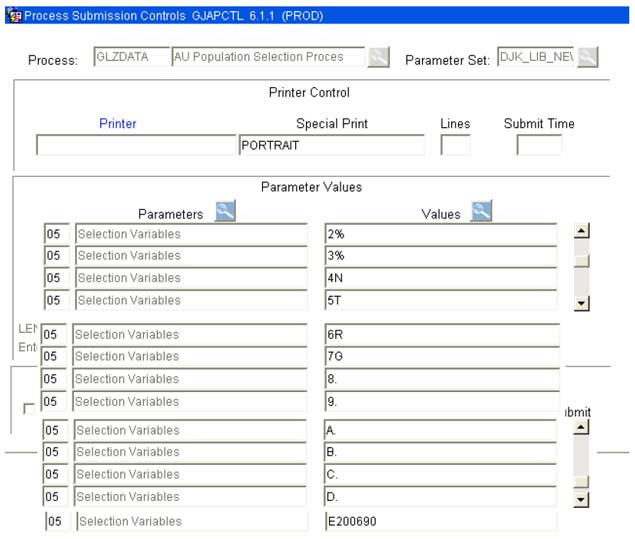# GLZDATA Population Selection

This is the procedure using GLZDATA population selection with numerous values.• In GLZDATA, if you have a number of parameters that run over 9 variables, you can start to use the alphabet. See example above.
• You will number each value starting with 1 and then go thru 9 and then start with A
• If there is no value – you can use a period